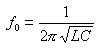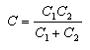# Colpitts Oscillator Calculator

Colpitts oscillator circuit is shown in Figure 1, Q is the transistor. The Colpitts and Hartley oscillator circuit are similar, except the inductor, capacitor exchange of positions. Oscillator equivalent circuit shown in Figure 2.Figure 1Figure 2

Capacitors C1, C2, and inductor L form a positive feedback network of selected frequency, feedback signals from both ends of capacitor C2, so called three-points capacitor oscillator circuit, also known as the feedback capacitor oscillator circuit. Feedback signal in phase with the input voltage to meet the oscillation of the phase equilibrium conditions, LC resonant circuit Q value is high enough under the conditions of the circuit's oscillation frequency is approximately equal to the resonant frequency circuit. Calculated as follows:AmongCapacitance three-point oscillator circuit is characterized by a high oscillation frequency can do in general can reach more than 100MHz, because of the high harmonic impedance of C2 is small, the feedback voltage in the high harmonic components smaller, and thus oscillation waveform better . Also when the oscillation frequency is high, C1, C2's value is very small, transistor-level capacitance will impact on the frequency.

With this tool, you can:

Enter the value of capacitance and the inductance to calculate frequency

 Capacitor C1 pFnFuF Capacitor C2 pFnFuF Inductor L nHuHmH Frequency f HzKHzMHz

Enter the value of capacitance and frequency to calculate inductance:

 Capacitor C1 pFnFuF Capacitor C2 pFnFuF Frequency f HzKHzMHz Inductor L nHuHmH

More Related Pages